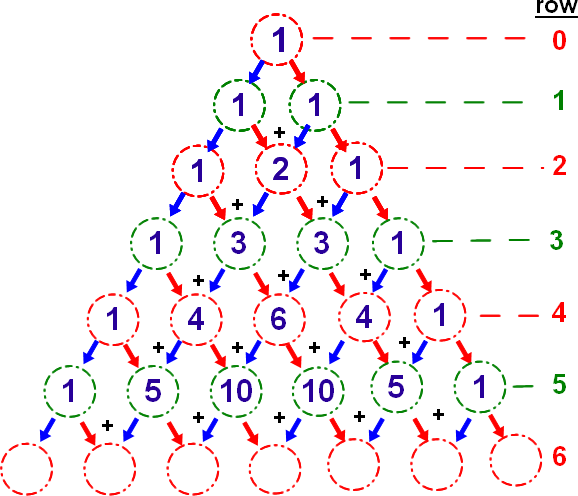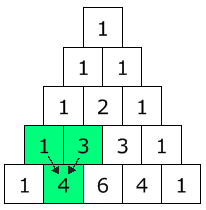### Lecture on Binomial TheoremThere are several closely related results that are variously known as the binomial theorem depending on the source. Even more confusingly a number of these (and other) related results are variously known as the binomial formula, binomial expansion. Binomial theorem describes the algebraic expansi.....

### Lecture on CombinationCombinations refer to different sets or groups made out of a given lot, without repeating an element, taking one or more of them at a time. If a number of things is given, each collection of things which can be made out of the above things is called  a combination.

.....

### Lecture on Basic Binomial TheoremBinomial Theorem describes the algebraic expansion of powers of a binomial. According to the theorem, it is possible to expand the power (x + y)n into a sum involving terms of the form axbyc, where the exponents b and c are nonnegative integers with b + c = n, and the coefficient a of each term.....